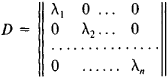# Symmetric Matrix

Also found in: Dictionary, Wikipedia.

## symmetric matrix

[sə′me·trik ′mā·triks]
(mathematics)
A matrix which equals its transpose.

## Symmetric Matrix

a square matrix S = ||sik|| in which any two elements that are symmetrically located with respect to the principal diagonal are equal: sik = ski, where i, k = 1, 2,…, n. A symmetric matrix is often treated as the matrix of the coefficients of some quadratic form. The theory of symmetric matrices and the theory of quadratic forms are closely related.

The properties of the spectrum of a symmetric matrix with real elements include the following: (1) all the roots λ1, λ2,…, λn of the characteristic equation of the matrix are real; and (2) to these roots there correspond n pairwise orthogonal eigenvectors of the matrix, where n is the order of the matrix. A symmetric matrix with real elements can always be represented in the form S″ = ODO-1, where O is an orthogonal matrix andReferences in periodicals archive ?
Let A [member of] [R.sup.nxn] be a nonsingular symmetric matrix, and let V [member of] [R.sup.nxs].
(A1) There exist a symmetric matrix B and positive constants [delta] and [gamma] such that
while the symmetric matrix [E.sub.ji] is gauge-invariant and is the symmetric square-root of the metric [g.sub.ij].
It should be noted that [C.sup.T]C is a real symmetric matrix, the condition number is easy to obtain.
Let P [member of] [R.sup.(n-r)x(n-r)] be a nonzero symmetric matrix. For the symmetric matrix [mathematical expression not reproducible] [V.sup.T] we have [AX.sub.0] = [O.sub.m,n] and the proof of Lemma 3 is complete.
where [C.sub.[kappa]](X) is the zonal polynomial of mxm complex symmetric matrix X corresponding to the ordered partition [kappa] = ([k.sub.1], ..., [k.sub.m]), [k.sub.1] [greater than or equal to] ...
where [a.sub.i], i = 1, ..., p, [b.sub.j], j = 1, ..., q are arbitrary complex numbers, X is an m x m complex symmetric matrix, [C.sub.[kappa]](X) is the zonal polynomial of m x m complex symmetric matrix X corresponding to the ordered partition k = ([k.sub.1], ..., [k.sub.m]), [k.sub.1] [greater than or equal to] ...
SDP problems arise from the well-known linear programming problems by replacing the vector of variables with a symmetric matrix and replacing the non-negativity constraints with positive semidefinite constraints.
Theorem 2: A matrix [LAMBDA] is Hurwitz, that is, Re[[lambda].sub.i] [less than or equal to] 0 for all eigenvalues of [lambda], if and only if for any given positive-definite symmetric matrix Qthere a positive-definite symmetric matrix Pthat satisfies the Lyapunov equation
matrix C reduces to a positive definite symmetric matrix P; as a result, (10) reduces to (25).
The symmetric block component of a symmetric matrix is denoted by *.
The commonly used approach for spectral clustering link data is to obtain a symmetric matrix [bar.A] from the original adjacency A and then to apply spectral clustering techniques to [bar.A].

Site: Follow: Share:
Open / Close